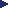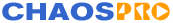HOMEFeaturesGalleryDownloadDocumentationResourcesMailing ListsProgramsFeature VotingWishlistTheoryBifurcationJulia/MandelD-SystemsLyapunovIFSL-SystemsPlasmaTutorialsFormula DBContactRelease 4.0### Dynamic Systems, Strange Attractors

In the year 1961 the meteorologist Edward Lorenz examined a system of a few differential equations, a system, where not concrete points are known, from which one can calculate another. Instead, only the derivation of each point is known, defined by a function, so that approximations for successing points can be calculated. (Please, forgive me for this explanation of differential equations...)

Well, he made experiments and found out, that his result heavily depend on the used numerical precision. An incredibly small change of the starting value caused a totally different result. So the title of one of his publications was: Kann das Flattern eines Schmetterlings in Brasilien einen Orkan in Texas verursachen? (in english: Can the fluttering of a butterfly in Brasil cause a hurricane in Texas?). The answer / his answer was yes. Since then on, this effect is called Schmetterlingseffekt (in english perhaps effect of a bufferfly). Edward Lorenz then greatly simplified his model in order to study it and to experiment with it. It contained only 3 differential equations, thus we can experiment with it, too:

dx/dt=-ax+ay
dy/dt=cx-y-xz
dz/dt=-bz+xy

Mr. Lorenz experimented mainly with a=10, b=8/3 and different values for c. The resulting object can be interpreted as a 3-dimensional curve and, if an initial point is given, it's from a mathematical point of view (theoretically) definite, but not in practice.

Mr. Lorenz then chose c=28 and calculated the curve for various initial points. But although the curve started totally different, he found, that after a few seconds always the same figure appeared. It had a very complicated structure, it was built from an infinite number of loops, and the whole thing was very strange... It looked like the 3 differential equations stamped a very complicated structure into the 3-dimensional world in his computer, a fractal attractor, which attracts every point in this world. Because the structure was so complicated, such an attractor is called strange attractor.

Last update on Nov 26 2002.